V.6 No 1 45 On the wave-particle dualityAccording to such supposition of Born, “we think the initial electron beam in association with the related de Broglie wave. Falling onto the atom, this wave originates the secondary spherical wave. Proceeding from the optical analogy, we expect that the quadratic form of a definite shape produced from the wave amplitude can be interpreted as the density of flow or, otherwise, the number of scattered electrons. When the related computations have been done (Ventzel, Gordon), it appeared that for scattering on the nucleus just the Rutherford formula is yielded” [2, p. 115].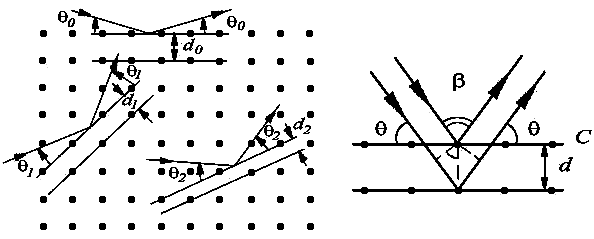Fig. 5. The scheme to found the Bragg – Wolf conditions for electron waves scattered on an ideal crystal
 In case of electron diffraction on an ideal crystal this calculation has the following form: “Electrons with the de Broglie wavelength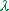B-V  can diffract on different atomic planes chosen by mutual orientation of falling electron beam and scattering crystal. Let electrons fall onto crystal with a sliding angle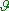with respect to the scattering set of planes. To simplify, consider a symmetric case (Fug. 5b) when the crystal surface C is parallel to the scattering planes, though this condition is kept far from always. Then the anglewill be the sliding angle under which the electrons fall onto the crystal surface, and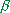=- 2will be the angle between the fallen and diffracted beams of electrons. Theoretically the analysis of electron diffraction on crystals is much similar to the case of X-rays. Atsatisfying the Bragg – Wolf condition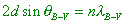(4)
 an intensive diffraction maximum of reflected wave arises. HereB-V  is the Bragg angle, d is the distance between the reflecting planes (the lattice constant), n is the integer number with the values 1, 2, 3, … named the order of reflection. The physical meaning of Bragg – Wolf condition (4) is quite transparent: the diffraction maximum appears in the cases when the difference of pace of waves reflected from the adjacent atomic planes is equal to the integer number of de Broglie wavelengths. Just in this case the reflected waves strengthen each other, i.e. the constructive interference takes place. Note that the condition (4) has been yielded without account of electron wave refraction in crystal. With account of refraction, the Bragg – Wolf condition will take the form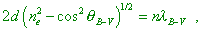(5)
 where ne  is the index of electron wave refraction … We can see the result of Davisson and Germer experimental studies in Fig. 6 where we show the polar diagrams of intensity of the reflected electrons at some values of the accelerating difference of potentials. At U = 44 V (Fig. 6a) the diffraction maximum only begins forming under an angle= 50o, at U  = 54 V (Fig. 6c) it reaches its maximal intensity, and in further growth of U (Fig. 6d, e) again falls to a full disappearance …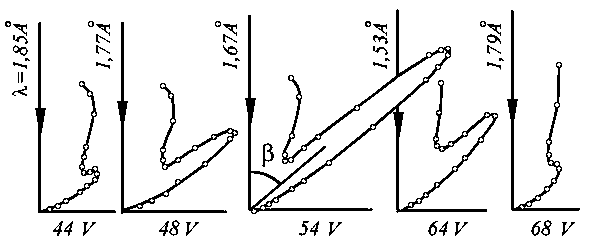Fig. 6. The dynamics of diffraction reflection of electrons at change of accelerating difference of potentials U
 The difference of theory and experiment was that the locations of diffraction maximums observed in the experiment did not coincide with the maximum locations determined after the Bragg – Wolf condition (4) and shown in Fig. 7 by vertical arrows. This difference was especially visual for little values, i.e. for a little value of the accelerating difference of potentials Un . The cause of such difference in the theory and experiment is that the Bragg – Wolf condition (4) disregards the refraction of electron waves in metal. The use of condition (5) fully cancels this discrepancy” .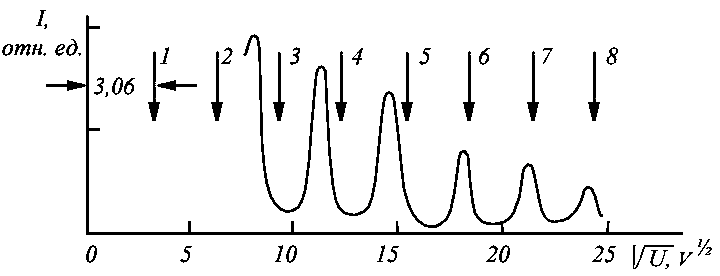Fig. 7. Dependence of the electron beam intensity diffracted on the nickel monocrystal on the accelerating strength U at the constant value of angle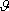With all outwardly matched derivation, we can note several points. First of all, as the authors of this citation admit, the theory agrees with experiment if using not the Bragg – Wolf formula but its modification that accounts the electron waves refraction on crystals. But if the authors of analogy in the de Broglie waves and X-rays base on the completeness of this analogy, the Bragg – Wolf formula for X-rays also has to be modified with account of refraction in crystal, as in this case “electric field of a falling wave ‘swings’ the charged particles (electrons) and the scattered radiation arises; in a rough approximation we can describe it by the relationship for a harmonic oscillator. In particular, it becomes clear why the short-wave radiation is scattered most intensively” [6, p. 282]. But the Bragg – Wolf formula well describes the interference (not diffraction!) of X-rays in its initial form (4). “Factually, the reflection occurs from many planes, i.e. not two but many beams interfere with each other. However the influence of these multiple reflections does not change the conditions of maximum of interference but, the same as in optics, is revealed only in that instead the broad interference fringes we yield narrow lines, i.e. it appears very advantageous to apply this method in spectroscopy of X-rays. And the condition (4) for reflection remains unchanged” [1, p. 124]. Consequently, this discrepancy says again, the authors of quantum-wave conception did not account some crucial factors but substituted them by a simple adjusting of results. In case of condition (5) for X-rays the Bragg – Wolf formula will be discrepant with the experiment, as well as it will be discrepant with the measurement of the lattice constant with other methods. Thereupon, the value of lattice constant d will change and this will make again the discrepancy between the experiment and theory for electron de Broglie waves.   Noting the said, to resolve the wave-particle paradox becomes a crucial problem on whose solution depends, in which direction our understanding of physical processes in the micro-world will tend. The importance of this problem appeared the main reason to undertake the study whose results we will state in this paper.

Contents: / 43 / 44 / 45 / 46 / 47 / 48 / 49 / 50 / 51 / 52 /